# Plotting Seasonality and Correlation

timetk: A toolkit for time series analysis in R

This tutorial focuses on 3 new functions for visualizing time series diagnostics:

• ACF Diagnostics: `plot_acf_diagnostics()`
• Seasonality Diagnostics: `plot_seasonal_diagnostics()`
• STL Diagnostics: `plot_stl_diagnostics()`
``````library(tidyverse)
library(timetk)

# Setup for the plotly charts (# FALSE returns ggplots)
interactive <- FALSE``````

# Correlation Plots

## Grouped ACF Diagnostics

``````m4_hourly %>%
group_by(id) %>%
plot_acf_diagnostics(
date, value,               # ACF & PACF
.lags = "7 days",          # 7-Days of hourly lags
.interactive = interactive
)``````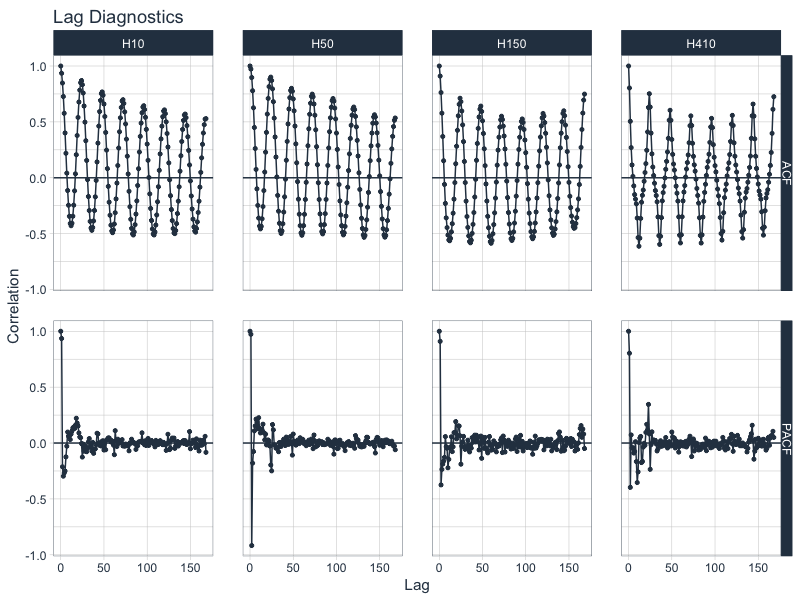## Grouped CCF Plots

``````walmart_sales_weekly %>%
select(id, Date, Weekly_Sales, Temperature, Fuel_Price) %>%
group_by(id) %>%
plot_acf_diagnostics(
Date, Weekly_Sales,        # ACF & PACF
.ccf_vars    = c(Temperature, Fuel_Price),   # CCFs
.lags        = "3 months",    # 3 months of weekly lags
.interactive = interactive
)``````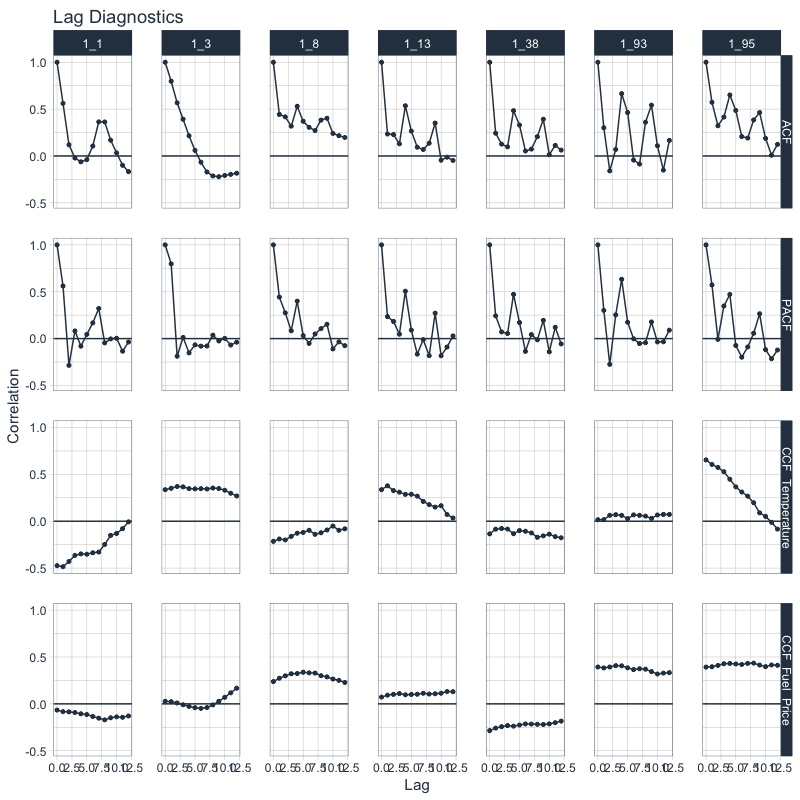# Seasonality

## Seasonal Visualizations

``````taylor_30_min %>%
plot_seasonal_diagnostics(date, value, .interactive = interactive)``````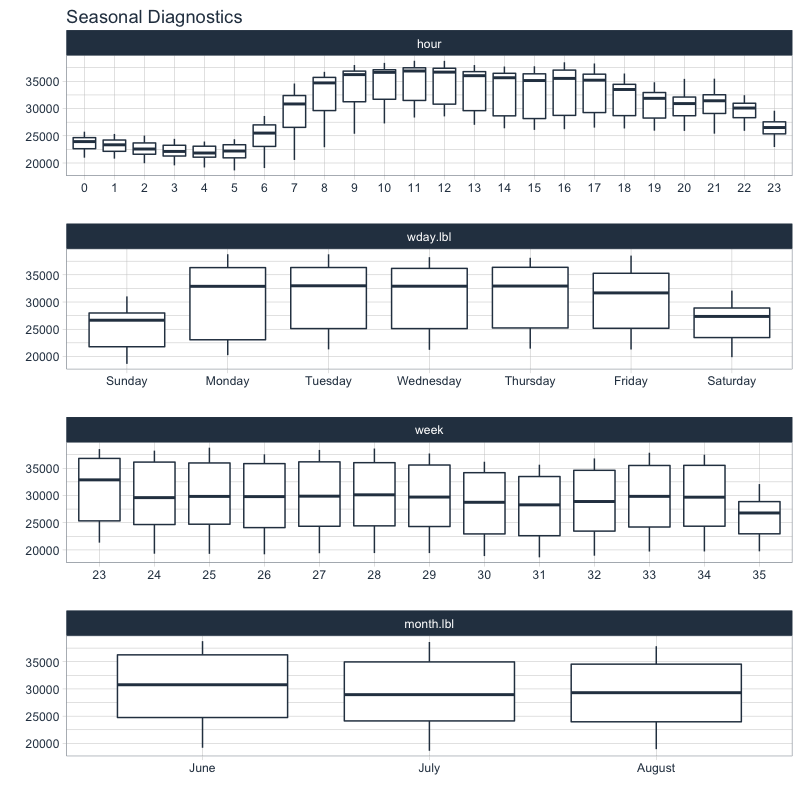## Grouped Seasonal Visualizations

``````m4_hourly %>%
group_by(id) %>%
plot_seasonal_diagnostics(date, value, .interactive = interactive)``````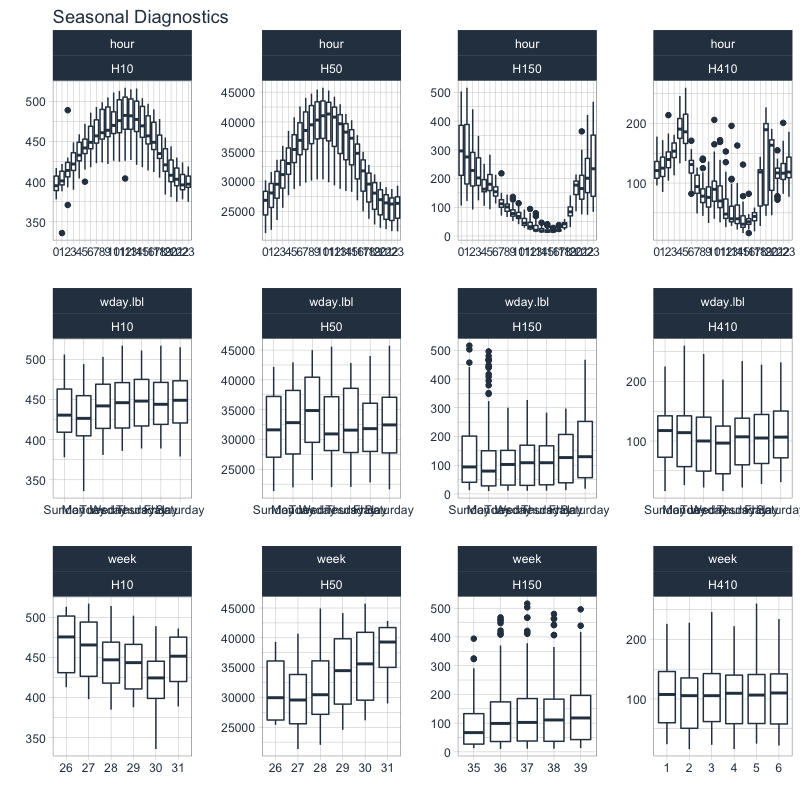# STL Diagnostics

``````m4_hourly %>%
group_by(id) %>%
plot_stl_diagnostics(
date, value,
.frequency = "auto", .trend = "auto",
.feature_set = c("observed", "season", "trend", "remainder"),
.interactive = interactive)``````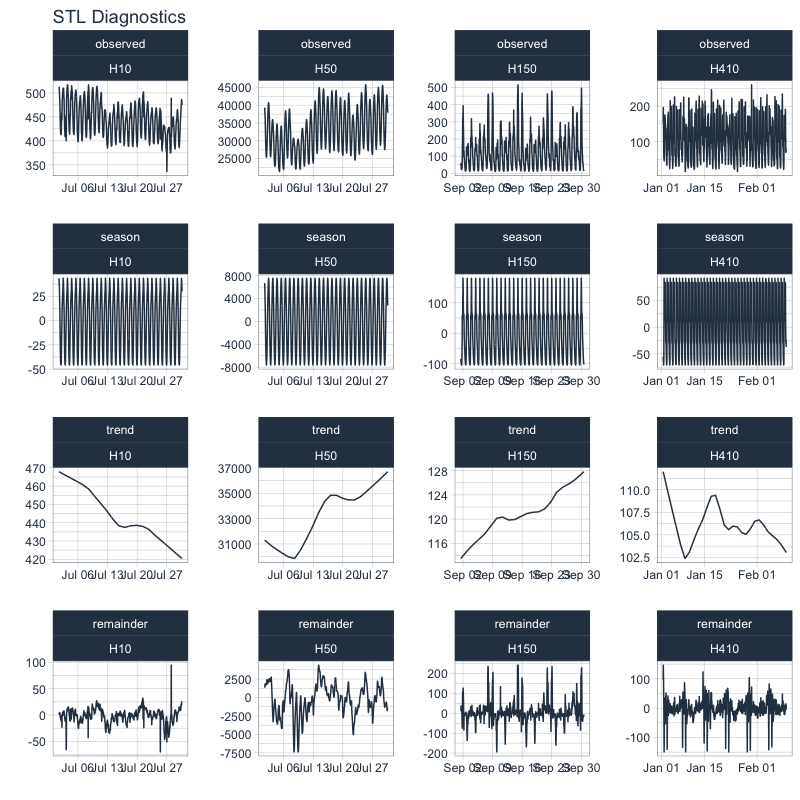# Learning More

If you are interested in learning from my advanced Time Series Analysis & Forecasting Course, then join my waitlist. The course is coming soon.